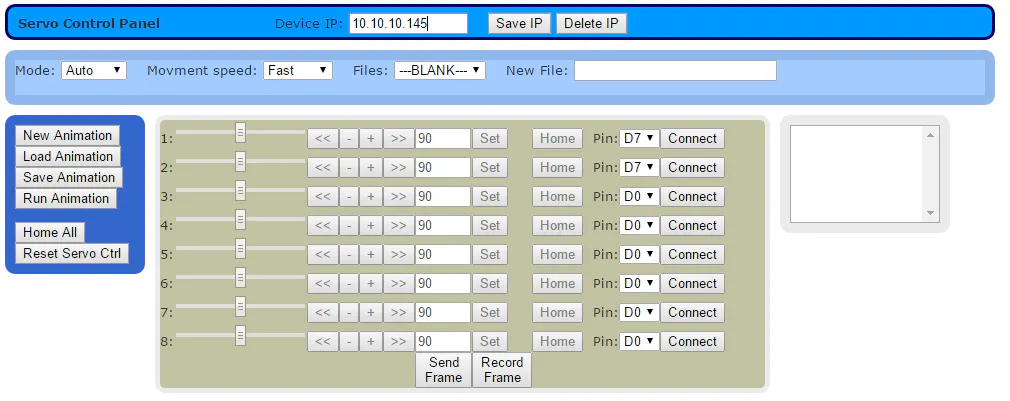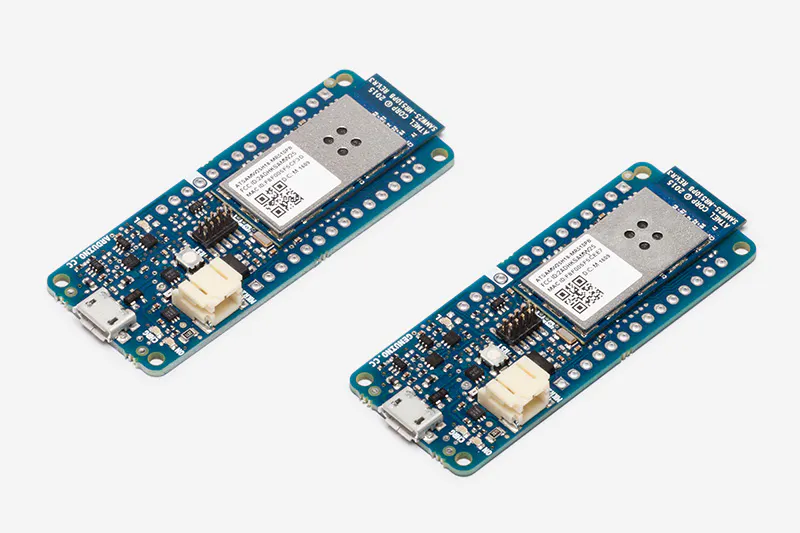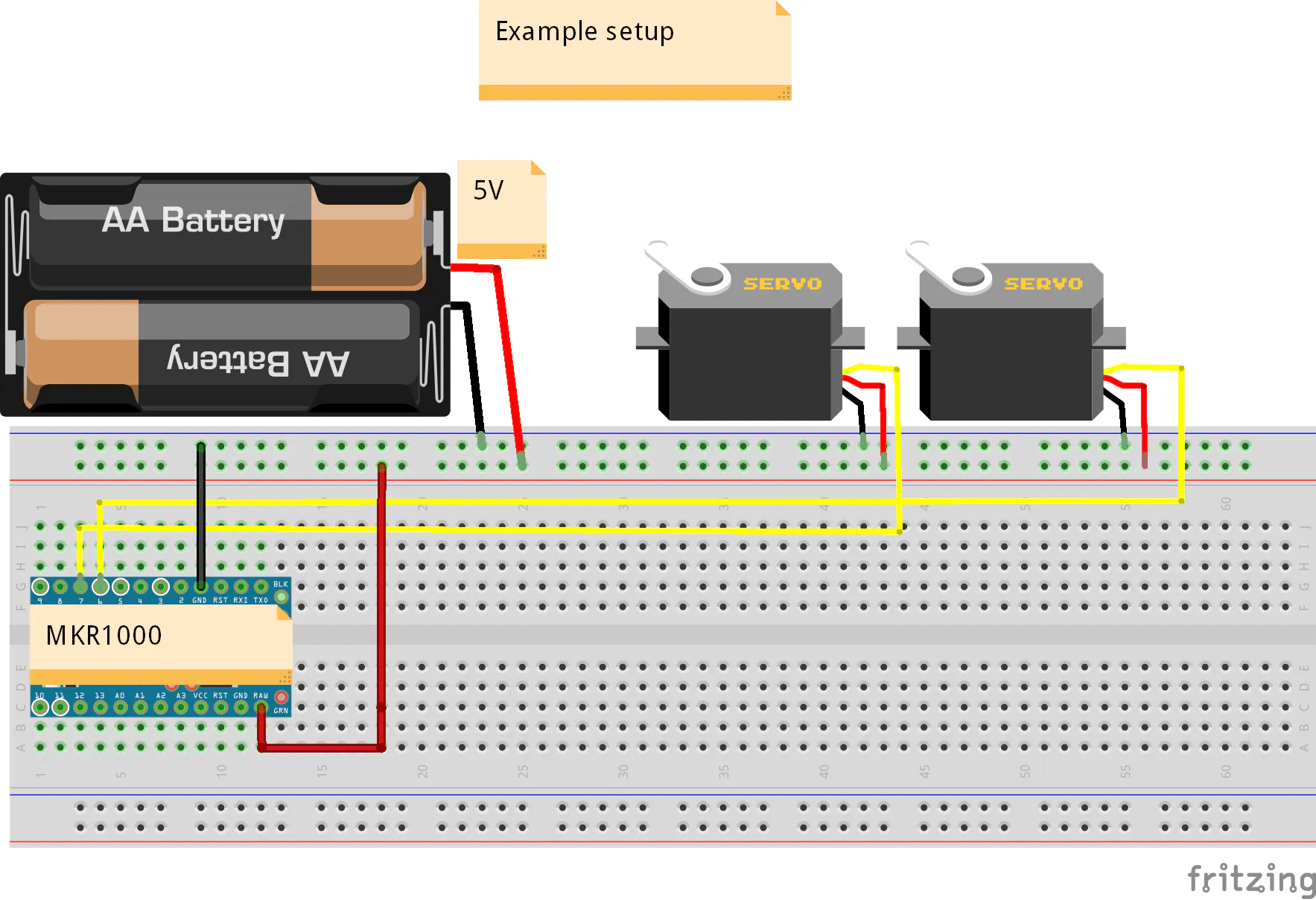# MKR1000 Servo Control Panel

A servo controller using the built-in WiFi abilities of the Arduino MKR1k. The panel consists of a MKR1k web listener and Javascript/ajax.

BeginnerFull instructions provided1 hour4,791## Things used in this project

### Hardware componentsArduino MKR1000
×1
 Servos (Tower Pro MG996R)
×1×1

### Software apps and online servicesArduino IDE

## Schematics

### Example servo setup## Code

### MKR1k Servo web responder

Arduino
```/*
Mkr1000-Servo control web responder

Goal in life:
Control servos via http get requests.

Written By - Scott Beasley 2016.
*/

#include <SPI.h>
#include <WiFi101.h>
#include <Servo.h>
#include <string.h>
#include <ctype.h>

void ServoOpen (String pin);
void ServoClose (String command);
void ServoSet (String command);
void ServoCtrlReset (void);
void sendReturnResp (WiFiClient client, String ret_data);
bool request_is (String request, String reqdata);

// Define your Wifi info here

#define BOARDNAME "mkr1000_01"

// Define to get extra info from the Serial port
#define DEBUG

// Globals
WiFiServer server (80);

// Allow for at least 8 servos.
struct userservos {
Servo servo;
int pin;
} userservos;
int servosinuse = 0;

String json_ret = "";

void setup (void)
{
servosinuse = 0;
int status = WL_IDLE_STATUS;
int i = 0;
Serial.begin (9600);
while (!Serial) {if (i++ >= 1024) break;}

// check for the presence of the shield:
if (WiFi.status ( ) == WL_NO_SHIELD) {
Serial.println ("WiFi shield not present");
// don't continue:
while (true);
}

// Mark all servo slots as free on start.
for (int i = 0; i < 9; i++) {
userservos[i].pin = -1;
}

// Connect to the WiFi network
Serial.print ("\nConnecting to: ");
Serial.println (SSID);

while (status != WL_CONNECTED) {
// Connect to WPA/WPA2 network. Change this line if using open or WEP network:
status = WiFi.begin(SSID, PASSWD);

// wait 10 seconds for connection:
delay (10000);
}

Serial.println ("\nWiFi connected");

// Start the tcp listener
server.begin ( );

IPAddress ip = WiFi.localIP ( );
Serial.print ("Use this URL to connect: ");
Serial.print ("http://");
Serial.print (ip);
Serial.println ("/");
}

void loop ( )
{
bool endofline = false;
json_ret = "";

WiFiClient client = server.available ( );

if (client) {
#ifdef DEBUG
Serial.println ("Client connected");
#endif
String currentLine = "";
while (client.connected ( )) {
if (client.available ( )) {
char c = client.read ( );
if (c == '\n') {
endofline = true;
} else if (c != '\r') {
currentLine += c;
}

if (endofline) {
endofline = false;
String parms = "";

#ifdef DEBUG
Serial.println ("<" + currentLine + ">");
#endif

// Copy the parms sent in the request.
int ndx1 = currentLine.indexOf ('?');
int ndx2 = currentLine.indexOf (' ', ndx1);

#ifdef DEBUG
Serial.println ("<" + String (ndx1) + ">");
Serial.println ("<" + String (ndx2) + ">");
#endif

if (ndx1 >= 0) {
parms = currentLine.substring (ndx1 + 1, ndx2);
}

#ifdef DEBUG
if (parms.length ( ) > 0)
Serial.println ("<" + parms + ">");
#endif

if (request_is (currentLine, "/servoOpen")){
ServoOpen (parms);
} else if (request_is (currentLine, "/servoClose")) {
ServoClose (parms);
} else if (request_is (currentLine, "/servoSet")) {
ServoSet (parms);
} else if (request_is (currentLine, "/servoRead")) {
} else if (request_is (currentLine, "/servoCtrlReset")) {
ServoCtrlReset ( );
json_ret = "{\n\t\"return_code\": 0\n}";
} else {
break;
}

#ifdef DEBUG
Serial.println ("<=" + json_ret + "=>");
#endif

sendReturnResp (client, json_ret);
currentLine = "";
break;
}
}
}

// Close the connection when done
client.stop ( );
#ifdef DEBUG
Serial.println ("Client disconnected normally");
#endif
}
}

bool request_is (String request, String reqdata)
{
return (request.indexOf (reqdata) != -1 ? 1 : 0);
}

void sendReturnResp (WiFiClient client, String ret_data)
{
String return_hdr = "HTTP/1.1 200 OK\r\n"
"Content-Type: application/json\r\n"
"Access-Control-Allow-Origin: *\r\n"
"Connection: close\r\n"
"Content-Length: " + String (ret_data.length ( )) +
"\r\n\r\n" + ret_data +"\r\n";
client.print (return_hdr);
}

void ServoOpen (String pin)
{
int i, return_code = 0;

//convert ascii to integer
int pinNumber = pin.charAt (0) - '0';
//Sanity check to see if the pin numbers are within limits
if (pinNumber < 0 || pinNumber > 9) return_code = -1;

servosinuse++;
if (servosinuse > 8) {
return_code = -3; // All servos inuse.
}

for (i = 0; i < 9; i++) {
if (userservos[i].pin == -1) {
// Zap any old attachment.
userservos[i].servo.detach ( );

userservos[i].pin = pinNumber;
userservos[i].servo.attach (pinNumber);
break;
}
}

// Return the servo array element index used.
json_ret = "{\n\t\"data_value\": \"" + String (i) +
"\",\n\t\"return_code\": " + return_code + "\n}";
}

void ServoClose (String command)
{
int return_code = 0;

//convert ascii to integer
int indexNumber = command.charAt(0) - '0';
//Sanity check to see if the element numbers are within limits
if (indexNumber < 0 || indexNumber > 9) return_code = -1;

userservos[indexNumber].pin = -1;
servosinuse--;
userservos[indexNumber].servo.detach ( );

json_ret = "{\n\t\"return_code\": " + String (return_code) + "\n}";
}

void ServoSet (String command)
{
int ret = -1;
//convert ascii to integer
int indexNumber = command.charAt (0) - '0';
//Sanity check to see if the pin numbers are within limits
if (indexNumber< 0 || indexNumber > 8) {
ret = -1;
} else {
String value = command.substring (2);
ret = 0;
userservos[indexNumber].servo.write (value.toInt ( ));
}

json_ret = "{\n\t\"return_code\": " + String (ret) + "\n}";
}

{
int return_code = 0;
int raw_val = 0;

//convert ascii to integer
int indexNumber = command.charAt (0) - '0';
//Sanity check to see if the pin numbers are within limits
if (indexNumber < 0 || indexNumber > 8) return_code = -1;

json_ret = "{\n\t\"data_value\": \"" + String (raw_val) +
"\",\n\t\"return_code\": " + String (return_code) + "\n}";
}

void ServoCtrlReset (void)
{
servosinuse = 0;
// Mark all servo slots as free and close any open.
for (int i = 0; i < 9; i++) {
if (userservos[i].pin != -1) {
userservos[i].servo.write (90); // Try and stop if moving.
userservos[i].servo.detach ( );
}

userservos[i].pin = -1;
}
}
```

### Servo Control panel Javascript/Ajax code.

HTML
```<!DOCTYPE html>
<!--
Mkr1000-Servo control panel

Goal in life:
Control servos.

Written By - Scott Beasley 2016.

CSS Box layout generated at http://www.cssportal.com
Public domain. Free to use or change. Enjoy :)
-->
<html>
<title>Servo Control Panel</title>
<script type="text/javascript">
var DEVICE_IP = '';
var running_ani = false;
{
return "Are you sure you wish to leave the Servo control panel?";
}

function showValue (id, newValue)
{
\$('#range' + id).val (newValue);
if (\$('#mode').val ( ) == "AUTO") {
setServoVal (id);
}
}

function setIncVal (id)
{
var servo_val;

servo_val = \$('#servo' + id).val ( );
servo_val++;
if (servo_val > 179) {
servo_val = 179;
}

\$('#range' + id).val (servo_val);
\$('#servo' + id).val (servo_val);
}

function setDecVal (id)
{
var servo_val;

servo_val = \$('#servo' + id).val ( );
servo_val--;
if (servo_val < 0) {
servo_val = 0;
}

\$('#range' + id).val (servo_val);
\$('#servo' + id).val (servo_val);
}

function setMin (id)
{
\$('#range' + id).val (0);
\$('#servo' + id).val (0);
}

function setMax (id)
{
\$('#range' + id).val (179);
\$('#servo' + id).val (179);
}

function homeAll ( )
{
var wait = 0;

for (var i = 1; i < 9; i++) {
if (\$('#conn_state' + i).val ( ) == "1") {
\$(window).delay(wait).queue (function ( ) {
homeServo (i, '90');
wait = 100;
});
}
}
}

function seeIfOpen (pin)
{
var ret = 0

for (var i = 1; i < 8; i++) {
if (\$('#conn_state' + i).val ( ) == "1") {
if (pin == \$('#gpio' + i).val ( )) {
ret = 1;
}
}
}

return ret;
}

{
var file;

file = \$('#files').val ( );
if (file == "") {
\$('#files').focus ( );
return;
}

\$('#files').append ('<option value="' + file + '" selected="selected"> '
+ file + ' </option>');
}

function AniNew ( )
{
var file;

file = \$('#newfile').val ( );
if (file == "") {
alert ("Need file name to save to");
\$('#newfile').focus ( );
return;
}

\$('#files').append ('<option value="' + file + '" selected="selected"> '
+ file + ' </option>');
}

function AniSave ( )
{
var stow_line, stow_key;

\$('#frames option').each (function ( ) {
\$(this).val ( );
});
}

function AniRun ( )
{

}

function recordFrame ( )
{
var servo1_val, servo2_val, servo3_val, servo4_val;
var frame_line;

servo1_val = \$('#servo1').val ( );
servo2_val = \$('#servo2').val ( );
servo3_val = \$('#servo3').val ( );
servo4_val = \$('#servo4').val ( );

frame_line = servo1_val + ',' + servo2_val + ',' + servo3_val + ',' + servo4_val;
\$('#frames').append ('<option>' + frame_line + '</option>');
}

function sendFrame ( )
{
var servo1_val, servo2_val, servo3_val, servo4_val, speed;
var frame_line;

servo1_val = \$('#servo1').val ( );
servo2_val = \$('#servo2').val ( );
servo3_val = \$('#servo3').val ( );
servo4_val = \$('#servo4').val ( );
speed = \$('#speed').val ( );

frame_line = speed + ',' + servo1_val + ',' + servo2_val + ',' +
servo3_val + ',' + servo4_val;
}

function homeServo (id, val)
{
\$('#range' + id).val (val);
\$('#servo' + id).val (val);
if (\$('#mode').val ( ) == "AUTO") {
setServoVal (id);
}
}

function connServo (servo)
{
var conn_state = \$('#conn_state' + servo).val ( );
var pin = \$('#gpio' + servo).val ( );
if (conn_state == "0") {
if (seeIfOpen (pin) == 1) {
return;
}

var requestURL = "http://" + DEVICE_IP + "/servoOpen?" + pin;
\$.get (requestURL, function (data) {
\$('#servo_id' + servo).val (data.data_value);
\$('#range' + servo).prop("disabled", false);
\$('#servo' + servo).prop("disabled", false);
\$('#setmin_but' + servo).prop("disabled", false);
\$('#inc_but' + servo).prop("disabled", false);
\$('#dec_but' + servo).prop("disabled", false);
\$('#setmin_but' + servo).prop("disabled", false);
\$('#setmax_but' + servo).prop("disabled", false);
\$('#set_val' + servo).prop("disabled", false);
\$('#set_but' + servo).prop("disabled", false);
\$('#home_but' + servo).prop("disabled", false);
\$('#conn_state' + servo).val ("1");
\$('#gpio' + servo).prop("disabled", true);
\$('#conn_but' + servo).text ('Disconnect');
});
} else {
var servo_id = \$('#servo_id' + servo).val ( );
var requestURL = "http://" + DEVICE_IP + "/servoClose?" + servo_id;

\$.get (requestURL, function (data) {});

//             \$(window).delay (100).queue (function ( ) {
// Try and trun the servo off first.
// After the servo turn off, send the close request.
\$('#range' + servo).val ('90');
\$('#servo' + servo).val ('90');
setServoVal (servo);
\$('#servo_id' + servo).val ('-1');
\$('#range' + servo).prop("disabled", true);
\$('#servo' + servo).prop("disabled", true);
\$('#setmin_but' + servo).prop("disabled", true);
\$('#inc_but' + servo).prop("disabled", true);
\$('#dec_but' + servo).prop("disabled", true);
\$('#setmin_but' + servo).prop("disabled", true);
\$('#setmax_but' + servo).prop("disabled", true);
\$('#set_val' + servo).prop("disabled", true);
\$('#set_but' + servo).prop("disabled", true);
\$('#home_but' + servo).prop("disabled", true);
\$('#conn_state' + servo).val ("0");
\$('#gpio' + servo).prop("disabled", false);
\$('#conn_but' + servo).text ('Connect');
//			   });
}
}

function setServoVal (servo)
{
var servo_hnd = \$('#servo_id' + servo).val ( );
var servo_val = \$('#range' + servo).val ( );
if (servo_val < 0) {
servo_val = 0;
} else if (servo_val > 179) {
servo_val = 179;
}
\$('#range' + servo).val (servo_val);
\$('#servo' + servo).val (servo_val);
console.log ("Action = " + servo_hnd + " set val = " + servo_val);
var requestURL = "http://" + DEVICE_IP + "/servoSet?" + servo_hnd +
',' + servo_val;
\$.get (requestURL, function (data) {});
}

function saveKeys ( )
{
localStorage.setItem ("SERVO_CTRL_IO_DEV_ID", \$('#device').val ( ));
}

function deleteKeys ( )
{
localStorage.removeItem ("SERVO_CTRL_IO_DEV_ID");
}

function initIt ( )
{
for (var i = 1; i < 9; i++) {
\$('#range' + i).prop("disabled", true);
\$('#servo' + i).prop("disabled", true);
\$('#setmin_but' + i).prop("disabled", true);
\$('#inc_but' + i).prop("disabled", true);
\$('#dec_but' + i).prop("disabled", true);
\$('#setmin_but' + i).prop("disabled", true);
\$('#setmax_but' + i).prop("disabled", true);
\$('#set_val' + i).prop("disabled", true);
\$('#set_but' + i).prop("disabled", true);
\$('#home_but' + i).prop("disabled", true);
}

if (localStorage.getItem ("SERVO_CTRL_IO_DEV_ID") == null) {
\$('#device').focus ( );
} else {
DEVICE_IP = localStorage.getItem ("SERVO_CTRL_IO_DEV_ID");
\$('#device').val (DEVICE_IP);
}
}

function resetcontroller ( )
{
var requestURL = "http://" + DEVICE_IP + "/servoCtrlReset";
\$.get (requestURL, function (data) {});
}
</script>
type="text/javascript" charset="utf-8">
</script>
<div id="wrapper">
<table>
<tr>
<td><p><span style="font-weight:bold">Servo Control Panel</span></p></td>
<td>&nbsp;&nbsp;&nbsp;&nbsp;&nbsp;&nbsp;&nbsp;&nbsp;&nbsp;&nbsp;
&nbsp;&nbsp;&nbsp;&nbsp;&nbsp;&nbsp;&nbsp;&nbsp;&nbsp;
Device IP:
<input type="input" id="device" value="" maxlength="50" size="12" />
</td>
<td>&nbsp;&nbsp;&nbsp;
<button onclick="saveKeys ( )">Save IP</button>
<button onclick="deleteKeys ( )">Delete IP</button>
</td>
</tr>
</table>
</div>
</div>
<table class="outset">
<tr>
<td>
Mode:
<select id="mode">
<option value="MAN">Manual</option>
<option selected="selected" value="AUTO">Auto</option>
</td>
<td>
&nbsp;&nbsp;&nbsp;&nbsp;
</td>
<td>
Movment speed:
<select id="speed">
<option value="1">Fast</option>
<option value="2">Medium</option>
<option value="3">Slow</option>
</td>
<td>&nbsp;&nbsp;&nbsp;&nbsp;</td>
<td>Files:
<select id="files">
<option value="">---BLANK---</option>
</td>
<td>&nbsp;&nbsp;&nbsp;&nbsp;</td>
<td>New File:
<input type="input" id="newfile" value="" maxlength="32" size="24" />
</td>
</tr>
</table>
</div>
</div>
<div id="leftcolumnwrap">
<div id="leftcolumn">
<table>
<tr><td>
<button onclick="AniNew ( )">New Animation</button>
</td></tr>
<tr><td>
</td></tr>
<tr><td>
<button onclick="AniSave ( )">Save Animation</button>
</td></tr>
<tr><td>
<button onclick="AniRun ( )">Run Animation</button>
</td></tr>
<tr><td>&nbsp;&nbsp;&nbsp;&nbsp;</td></tr>
<tr><td>
<button onclick="homeAll ( )">Home All</button>
</td></tr>
<tr><td>
<button onclick="resetcontroller ( )">Reset Servo Ctrl</button>
</td></tr>
</table>
</div>
</div>
<div id="contentwrap">
<div id="content">
<table>
<tr>
<td>
1:
</td>
<td>
<input id="servo1" type="range" min="0" max="179" value="90" onchange="showValue ('1', this.value)" />
</td>
<td>
<button id="setmin_but1" onclick="setMin ('1')"><<</button>
</td>
<td>
<button id="dec_but1" onclick="setDecVal ('1')">-</button>
</td>
<td>
<button id="inc_but1" onclick="setIncVal ('1')">+</button>
</td>
<td>
<button id="setmax_but1" onclick="setMax ('1')">>></button>
</td>
<td>
<input type="input" id="range1" value="90" maxlength="3" size="3" />
</td>
<td>
<button id="set_but1" onclick="setServoVal ('1')">Set</button>
</td>
<td>
<button id="home_but1" onclick="homeServo ('1', '90')">Home</button>
</td>
<td>
<input type="hidden" id="servo_id1" value="0" />
<input type="hidden" id="conn_state1" value="0" />
</td>
<td>
&nbsp;&nbsp;Pin:
</td>
<td>
<select id="gpio1">
<option value="0">D0</option>
<option value="1">D1</option>
<option value="2">D2</option>
<option value="3">D3</option>
<option value="4">D4</option>
<option value="5">D5</option>
<option value="6">D6</option>
<option value="7">D7</option>
<option value="8">D8</option>
</td>
<td>
<button id="conn_but1" onclick="connServo ('1')">Connect</button>
</td>
<td>&nbsp;&nbsp;&nbsp;&nbsp;&nbsp;&nbsp;&nbsp;&nbsp;
</td>
</tr>
<tr>
<td>
2:
</td>
<td>
<input id="servo2" type="range" min="0" max="179" value="90" onchange="showValue ('2', this.value)" />
</td>
<td>
<button id="setmin_but2" onclick="setMin ('2')"><<</button>
</td>
<td>
<button id="dec_but2" onclick="setDecVal ('2')">-</button>
</td>
<td>
<button id="inc_but2" onclick="setIncVal ('2')">+</button>
</td>
<td>
<button id="setmax_but2" onclick="setMax ('2')">>></button>
</td>
<td>
<input type="input" id="range2" value="90" maxlength="3" size="3" />
</td>
<td>
<button id="set_but2" onclick="setServoVal ('2')">Set</button>
</td>
<td>
<button id="home_but2" onclick="homeServo ('2', '90')">Home</button>
</td>
<td>
<input type="hidden" id="servo_id2" value="0" />
<input type="hidden" id="conn_state2" value="0" />
</td>
<td>
&nbsp;&nbsp;Pin:
</td>
<td>
<select id="gpio2">
<option value="0">D0</option>
<option value="1">D1</option>
<option value="2">D2</option>
<option value="3">D3</option>
<option value="4">D4</option>
<option value="5">D5</option>
<option value="6">D6</option>
<option value="7">D7</option>
<option value="8">D8</option>
</td>
<td>
<button id="conn_but2" onclick="connServo ('2')">Connect</button>
</td>
<td>&nbsp;&nbsp;&nbsp;&nbsp;&nbsp;&nbsp;&nbsp;&nbsp;
</td>
</tr>
<tr>
<td>
3:
</td>
<td>
<input id="servo3" type="range" min="0" max="179" value="90" onchange="showValue ('3', this.value)" />
</td>
<td>
<button id="setmin_but3" onclick="setMin ('3')"><<</button>
</td>
<td>
<button id="dec_but3" onclick="setDecVal ('3')">-</button>
</td>
<td>
<button id="inc_but3" onclick="setIncVal ('3')">+</button>
</td>
<td>
<button id="setmax_but3" onclick="setMax ('3')">>></button>
</td>
<td>
<input type="input" id="range3" value="90" maxlength="3" size="3" />
</td>
<td>
<button id="set_but3" onclick="setServoVal ('3')">Set</button>
</td>
<td>
<button id="home_but3" onclick="homeServo ('3', '90')">Home</button>
</td>
<td>
<input type="hidden" id="servo_id3" value="0" />
<input type="hidden" id="conn_state3" value="0" />
</td>
<td>
&nbsp;&nbsp;Pin:
</td>
<td>
<select id="gpio3">
<option value="0">D0</option>
<option value="1">D1</option>
<option value="2">D2</option>
<option value="3">D3</option>
<option value="4">D4</option>
<option value="5">D5</option>
<option value="6">D6</option>
<option value="7">D7</option>
<option value="8">D8</option>
</td>
<td>
<button id="conn_but3" onclick="connServo ('3')">Connect</button>
</td>
<td>&nbsp;&nbsp;&nbsp;&nbsp;&nbsp;&nbsp;&nbsp;&nbsp;
</td>
</tr>
<tr>
<td>
4:
</td>
<td>
<input id="servo4" type="range" min="0" max="179" value="90" onchange="showValue ('4', this.value)" />
</td>
<td>
<button id="setmin_but4" onclick="setMin ('4')"><<</button>
</td>
<td>
<button id="dec_but4" onclick="setDecVal ('4')">-</button>
</td>
<td>
<button id="inc_but4" onclick="setIncVal ('4')">+</button>
</td>
<td>
<button id="setmax_but4" onclick="setMax ('4')">>></button>
</td>
<td>
<input type="input" id="range4" value="90" maxlength="3" size="3" />
</td>
<td>
<button id="set_but4" onclick="setServoVal ('4')">Set</button>
</td>
<td>
<button id="home_but4" onclick="homeServo ('4', '90')">Home</button>
</td>
<td>
<input type="hidden" id="servo_id4" value="0" />
<input type="hidden" id="conn_state4" value="0" />
</td>
<td>
&nbsp;&nbsp;Pin:
</td>
<td>
<select id="gpio4">
<option value="0">D0</option>
<option value="1">D1</option>
<option value="2">D2</option>
<option value="3">D3</option>
<option value="4">D4</option>
<option value="5">D5</option>
<option value="6">D6</option>
<option value="7">D7</option>
<option value="8">D8</option>
</td>
<td>
<button id="conn_but4" onclick="connServo ('4')">Connect</button>
</td>
<td>&nbsp;&nbsp;&nbsp;&nbsp;&nbsp;&nbsp;&nbsp;&nbsp;
</td>
</tr>
<tr>
<td>
5:
</td>
<td>
<input id="servo5" type="range" min="0" max="179" value="90" onchange="showValue ('5', this.value)" />
</td>
<td>
<button id="setmin_but5" onclick="setMin ('5')"><<</button>
</td>
<td>
<button id="dec_but5" onclick="setDecVal ('5')">-</button>
</td>
<td>
<button id="inc_but5" onclick="setIncVal ('5')">+</button>
</td>
<td>
<button id="setmax_but5" onclick="setMax ('5')">>></button>
</td>
<td>
<input type="input" id="range5" value="90" maxlength="3" size="3" />
</td>
<td>
<button id="set_but5" onclick="setServoVal ('5')">Set</button>
</td>
<td>
<button id="home_but5" onclick="homeServo ('5', '90')">Home</button>
</td>
<td>
<input type="hidden" id="servo_id5" value="0" />
<input type="hidden" id="conn_state5" value="0" />
</td>
<td>
&nbsp;&nbsp;Pin:
</td>
<td>
<select id="gpio5">
<option value="0">D0</option>
<option value="1">D1</option>
<option value="2">D2</option>
<option value="3">D3</option>
<option value="4">D4</option>
<option value="5">D5</option>
<option value="6">D6</option>
<option value="7">D7</option>
<option value="8">D8</option>
</td>
<td>
<button id="conn_but5" onclick="connServo ('5')">Connect</button>
</td>
<td>&nbsp;&nbsp;&nbsp;&nbsp;&nbsp;&nbsp;&nbsp;&nbsp;
</td>
</tr>
<tr>
<td>
6:
</td>
<td>
<input id="servo6" type="range" min="0" max="179" value="90" onchange="showValue ('6', this.value)" />
</td>
<td>
<button id="setmin_but6" onclick="setMin ('6')"><<</button>
</td>
<td>
<button id="dec_but6" onclick="setDecVal ('6')">-</button>
</td>
<td>
<button id="inc_but6" onclick="setIncVal ('6')">+</button>
</td>
<td>
<button id="setmax_but6" onclick="setMax ('6')">>></button>
</td>
<td>
<input type="input" id="range6" value="90" maxlength="3" size="3" />
</td>
<td>
<button id="set_but6" onclick="setServoVal ('6')">Set</button>
</td>
<td>
<button id="home_but6" onclick="homeServo ('6', '90')">Home</button>
</td>
<td>
<input type="hidden" id="servo_id6" value="0" />
<input type="hidden" id="conn_state6" value="0" />
</td>
<td>
&nbsp;&nbsp;Pin:
</td>
<td>
<select id="gpio6">
<option value="0">D0</option>
<option value="1">D1</option>
<option value="2">D2</option>
<option value="3">D3</option>
<option value="4">D4</option>
<option value="5">D5</option>
<option value="6">D6</option>
<option value="7">D7</option>
<option value="8">D8</option>
</td>
<td>
<button id="conn_but6" onclick="connServo ('6')">Connect</button>
</td>
<td>&nbsp;&nbsp;&nbsp;&nbsp;&nbsp;&nbsp;&nbsp;&nbsp;
</td>
</tr>
<tr>
<td>
7:
</td>
<td>
<input id="servo7" type="range" min="0" max="179" value="90" onchange="showValue ('7', this.value)" />
</td>
<td>
<button id="setmin_but7" onclick="setMin ('7')"><<</button>
</td>
<td>
<button id="dec_but7" onclick="setDecVal ('7')">-</button>
</td>
<td>
<button id="inc_but7" onclick="setIncVal ('7')">+</button>
</td>
<td>
<button id="setmax_but7" onclick="setMax ('7')">>></button>
</td>
<td>
<input type="input" id="range7" value="90" maxlength="3" size="3" />
</td>
<td>
<button id="set_but7" onclick="setServoVal ('7')">Set</button>
</td>
<td>
<button id="home_but7" onclick="homeServo ('7', '90')">Home</button>
</td>
<td>
<input type="hidden" id="servo_id7" value="0" />
<input type="hidden" id="conn_state7" value="0" />
</td>
<td>
&nbsp;&nbsp;Pin:
</td>
<td>
<select id="gpio7">
<option value="0">D0</option>
<option value="1">D1</option>
<option value="2">D2</option>
<option value="3">D3</option>
<option value="4">D4</option>
<option value="5">D5</option>
<option value="6">D6</option>
<option value="7">D7</option>
<option value="8">D8</option>
</td>
<td>
<button id="conn_but7" onclick="connServo ('7')">Connect</button>
</td>
<td>&nbsp;&nbsp;&nbsp;&nbsp;&nbsp;&nbsp;&nbsp;&nbsp;
</td>
</tr>
<tr>
<td>
8:
</td>
<td>
<input id="servo8" type="range" min="0" max="179" value="90" onchange="showValue ('8', this.value)" />
</td>
<td>
<button id="setmin_but8" onclick="setMin ('8')"><<</button>
</td>
<td>
<button id="dec_but8" onclick="setDecVal ('8')">-</button>
</td>
<td>
<button id="inc_but8" onclick="setIncVal ('8')">+</button>
</td>
<td>
<button id="setmax_but8" onclick="setMax ('8')">>></button>
</td>
<td>
<input type="input" id="range8" value="90" maxlength="3" size="3" />
</td>
<td>
<button id="set_but8" onclick="setServoVal ('8')">Set</button>
</td>
<td>
<button id="home_but8" onclick="homeServo ('8', '90')">Home</button>
</td>
<td>
<input type="hidden" id="servo_id8" value="0" />
<input type="hidden" id="conn_state8" value="0" />
</td>
<td>
&nbsp;&nbsp;Pin:
</td>
<td>
<select id="gpio8">
<option value="0">D0</option>
<option value="1">D1</option>
<option value="2">D2</option>
<option value="3">D3</option>
<option value="4">D4</option>
<option value="5">D5</option>
<option value="6">D6</option>
<option value="7">D7</option>
<option value="8">D8</option>
</td>
<td>
<button id="conn_but8" onclick="connServo ('8')">Connect</button>
</td>
<td>&nbsp;&nbsp;&nbsp;&nbsp;
</td>
</tr>
<tr>
<td>			</td>	<td>			</td>
<td>			</td>	<td>			</td>
<td>			</td>	<td>			</td>
<td>
<button onclick="sendFrame ( )">Send Frame</button>
</td>
<td>
<button onclick="recordFrame ( )">Record Frame</button>
</td>
</tr>
</table>
</div>
</div>
<div id="content2wrap">
<div id="content2">
<select id="frames" size="6" width="150" style="width: 150px">
</div>
</div>
</div>
</body>
</html>
```

## Credits

### Scott Beasley

9 projects • 55 followers
Well odd